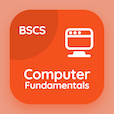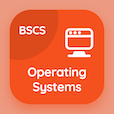Computer Science Courses Online

Chapter 4: Digital Logic Design Exam Tests

Digital Logic Design MCQs - Chapter 4

# Binary Systems Multiple Choice Questions (MCQ Quiz) PDF - 1

The Book Binary Systems Multiple Choice Questions and Answers (MCQs) PDF, Binary Systems quiz answers, test 1 to study online Digital Logic Design Course. Practice Binary Code MCQs, Binary Systems trivia questions and answers PDF to prepare for job interview. The eBook Binary Systems MCQs App Download: logic gates in digital logic design, definition of binary logic, subtraction with complement career test for associates in computer science.

The Multiple Choice Question (MCQ Quiz): Binary coded decimal is a combination of PDF, "Binary Systems" App Download (Free) with three binary digits, two binary digits, four binary digits, and five binary digits choices for top computer science schools. Solve binary code quiz questions, download Google eBook (Free Sample) for online degrees.

## Logic Design: Binary Systems MCQs Questions

MCQ: Binary coded decimal is a combination of

A) two binary digits
B) three binary digits
C) four binary digits
D) five binary digits

MCQ: Electronic digital circuits are also known as

A) Switching algebra
B) Logic circuits
C) Binary circuits
D) Binary algebra

MCQ: Which of the following gate is represented by x+y=z?

A) NOR gate
B) OR gate
C) NOT gate
D) XOR gate

MCQ: Using 10's complement subtracting 3250 from 72532 is equal to

A) 69282
B) 69272
C) 69252
D) 69232

MCQ: How many bits would be required to encode decimal numbers 0 to 9999 in straight binary codes?

A) 12
B) 14
C) 18
D) 16

Download Digital Logic Design Quiz App, Computer Fundamentals MCQ App, and Operating Systems MCQs App to install for Android & iOS devices. These Apps include complete analytics of real time attempts with interactive assessments. Download Play Store & App Store Apps & Enjoy 100% functionality with subscriptions!